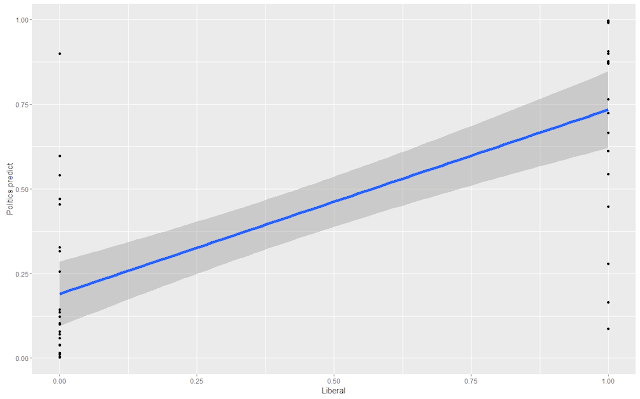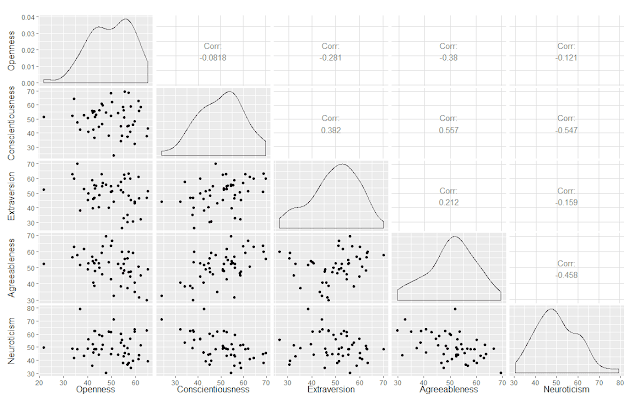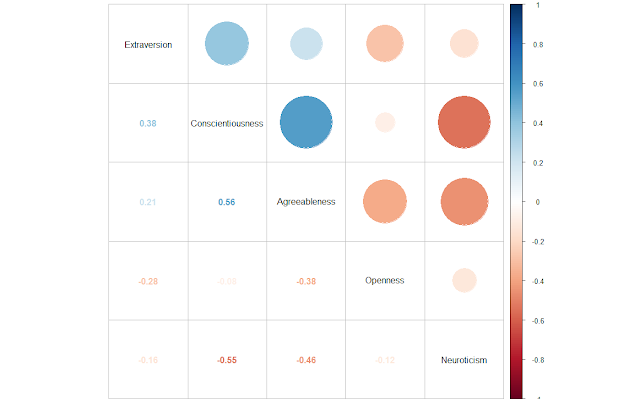### Neural Networks in R (Part 1 of 4) - Logistic Regression and neuralnet on State 'Personality' and Political Outcomes

This is an example of neural networks using the neuralnet package on one of my sample data sets, walking through a Pluralsight training series, Data Mining Algorithms in SSAS, Excel, and R.

In terms of results, the regression primarily predicts voter leanings based on five (5) traits, openness, conscientiousness, extraversion, agreeableness, and neuroticism, although only the first two (2) traits have a significant impact. Logistic regression is about 85% predictive, and slightly better at predicting Red states over its ability in predicting Blue states.

``````  "Logistic Regression (All) - Correct (%) = 0.854166666666667"
 "Logistic Regression (Red) - Correct (%) = 0.892857142857143"
 "Logistic Regression (Blue) - Correct (%) = 0.8"
``````

For the neuralnet package, I created a loop to vary the hidden layers and the number of repetitions, since this is such a small number of records. This is obviously much less predictive than logistic regression, but this seems to be a function of the package. In later experiments I use caret and nnet, and the results are generally better than logistic regression,

``````Layer Repetition  Prediction
1      1      0.6776156893
1      2      0.6776156893
1      3      0.6776156893
1      4      0.6776156893
1      5      0.6776156893
2      1      0.6776156893
2      2      0.6776156893
2      3      0.6776156893
2      4      0.6773258622
2      5      0.6880011593
``````

Also, as part of the neuralnet section, I explored correlations between some of the inputs, as it seems that excluding redundant or uncorrelated values might be useful.

The code is below, as are some related graphs. Source data is here.

``````
################################################
# Neural Net - Big Five By State
#
# Data Mining Algorithms in SSAS, Excel, and R
# https://app.pluralsight.com/player?course=data-mining-algorithms-ssas-excel-r&author=dejan-sarka&name=data-mining-algorithms-ssas-excel-r-m2
#
################################################

################################################
* prep workspace
################################################

# Clear memory
rm(list = ls())

# update all packages

################################################
################################################

# set Working directory
getwd()
setwd('../Data')

Politics.df <- read.csv("BigFiveScoresByState.csv", na.strings = c("", "NA"))
nrow(Politics.df)

# remove NULLs
Politics.df <- na.omit(Politics.df)
nrow(Politics.df)

################################################
# create formula used for all samples
################################################

names(Portfolio.df)

equation <- as.formula("Liberal ~ Openness + Conscientiousness + Extraversion + Neuroticism + Agreeableness")

################################################
# Logisitc Regression
################################################

# generalized logistic model
Politics.logR <- glm(data = Politics.df, family = binomial(), equation)

# summary
#summary(Politics.logR)

# confidence interval
#confint(Politics.logR)

# predict
Politics.predict <- predict(Politics.logR, type = "response")

# combine frame for calculation
Politics.combined <- cbind(Politics.predict, Politics.df)

# visually review data frame result
#View(Politics.combined)

# calculate percent correct
Politics.combined\$predictionBinary <- ifelse(Politics.combined\$Politics.predict >= .5, 1, 0)
Politics.combined\$Correct <- ifelse(Politics.combined\$predictionBinary == Politics.combined\$Liberal, 1, 0)
(Correct.LogR <- paste("Logistic Regression (All) - Correct (%) = ", (sum(Politics.combined\$Correct) / length(Politics.combined\$Correct))))

# percent correct Red
Politics.combined.red <- Politics.combined[Politics.combined\$Liberal == 0,]
(Correct.LogR.Red <- paste("Logistic Regression (Red) - Correct (%) = ", (sum(Politics.combined.red\$Correct) / length(Politics.combined.red\$Correct))))

# percent correct Red
Politics.combined.blue <- Politics.combined[Politics.combined\$Liberal == 1,]
(Correct.LogR.Blue <- paste("Logistic Regression (Blue) - Correct (%) = ", (sum(Politics.combined.blue\$Correct) / length(Politics.combined.blue\$Correct))))

# graph log regression
library(ggplot2)
plotPart1 <- ggplot(data = Politics.combined, aes(y = Politics.predict, x = Liberal))
plotPart1 + geom_point() + stat_smooth(method = "lm", formula = y ~ x, size = 2)################################################
# Neural Net with neuralnet
################################################

install.packages('neuralnet')
library(neuralnet)

# chart correlations to select candidates for inclusion
Politics.corr <- as.data.frame(cbind(Politics.df\$Openness, Politics.df\$Conscientiousness, Politics.df\$Extraversion, Politics.df\$Agreeableness, Politics.df\$Neuroticism))
colnames(Politics.corr) <- c('Openness','Conscientiousness','Extraversion','Agreeableness','Neuroticism')

#install.packages("GGally", dependencies = TRUE)
library(GGally)
ggpairs(Politics.corr)#install.packages("corrplot", dependencies = TRUE)
library(corrplot)
corrplot.mixed(cor(Politics.corr), order = "hclust", tl.col = "black")# set parameters for neural net
maxLayers = 1
maxRep = 1
df.Size = maxLayers * maxRep

# create empty data frame, for best performance
NeuralNet.Predictions <- data.frame(Layer = numeric(df.Size), Repitition = numeric(df.Size), Prediction = numeric(df.Size), stringsAsFactors = FALSE)

# loop through parameters and neural net to build results
currentRow = 0
for (layerCount in 1:maxLayers) {
for (repCount in 1:maxRep) {

currentRow <- currentRow + 1
NeuralNet.Predictions\$Layer[currentRow] <- layerCount
NeuralNet.Predictions\$Repitition[currentRow] <- repCount

# train
Politics.nn <- neuralnet(equation, data = Politics.df, hidden = layerCount, rep = repCount)

# predict
Politics.prediction <- as.data.frame(prediction(Politics.nn))

# set comparison value
Politics.prediction\$predictionBinary <- ifelse(Politics.prediction\$rep1.Liberal >= .5, 1, 0)

# compare actual versus result
Politics.prediction\$Correct <- ifelse(Politics.prediction\$predictionBinary == Politics.prediction\$data.Liberal, 1, 0)

# find percent correct, add to array
correct <- (sum(Politics.prediction\$Correct) / length(Politics.prediction\$Correct))
NeuralNet.Predictions\$Prediction[currentRow] <- correct
}
}

# display results
NeuralNet.Predictions

# average / min / max prediction
(NeuralNet.Predictions.Correct <- paste("NeuralNet - Correct (%) = ", ave(NeuralNet.Predictions\$Prediction)))
(NeuralNet.Min <- paste("NeuralNet - Min (%) = ", min(NeuralNet.Predictions\$Prediction)))
(NeuralNet.Max <- paste("NeuralNet - Max (%) = ", max(NeuralNet.Predictions\$Prediction)))

# display last neural net
plot(Politics.nn)``````